# Khovanov Homology of Alternating Tangles

(diff) ← Older revision | Latest revision (diff) | Newer revision → (diff)
In Preparation

The information below is preliminary and cannot be trusted! (v)

Note added March 8, 2008. The problem this paperlet addresses remain interesting. The solution suggested here is probably false.

Joint with Hernando Burgos Soto.

The purpose of this paperlet is to state a generalization to the world of tangles of Lee's theorem [Lee] about the Khovanov homology of alternating links.

In a later paper Hernando Burgos Soto will define a certain category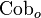$\operatorname{Cob}_o$ of "oriented cobordisms", in the same spirit as the category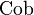$\operatorname{Cob}$ of . The objects of$\operatorname{Cob}_o$ are "oriented smoothing"; the orientations of the strands in an oriented smoothing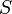$S$ allows us to define a certain integer parameter associated with it, its "rotation number"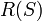$R(S)$. For degree-shifted smoothings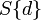$S\{d\}$, we further define a "degree-shifted" rotation number by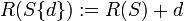$R(S\{d\}):=R(S)+d$. With these definitions we can state our two main theorems:

Theorem 1. Let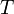$T$ be an alternating tangle. Then the Khovanov homology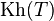$\operatorname{Kh}(T)$ of$T$ can be intepreted as a complex in the category$\operatorname{Cob}_o$ and within that category it is homotopy equivalent to a "one-diagonal" complex of the form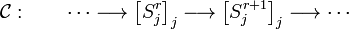${\mathcal C}:\qquad \cdots \longrightarrow \left[S^r_j\right]_j \longrightarrow \left[S^{r+1}_j\right]_j \longrightarrow \cdots$,

in which the homological degrees and the degree-shifted rotation numbers always lie along a single diagonal; i.e., for all smoothings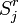$S^r_j$ in$\operatorname{Kh}(T)$ we have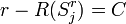$r-R(S^r_j)=C$,

where the constant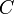$C$ is equal to ... Furthermore, all the differentials appearing in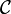${\mathcal C}$ are either simple saddles or "identity curtains with a single dot". (The precise definitions will appear in the forthcoming paper by Burgos Soto).

It is a simple matter to verify that in the case of alternating tangles with no boundary, i.e., in the case of alternating links, this theorem reduces to Lee's theorem on the Khovanov homology of alternating links.

We call the type of complexes appearing in Theorem 1 "on-diagonal complexes". Our second theorem is in fact the key to the proof of the first; for it reduces that proof to the simple task of verifying that the Khovanov homologies of the one-crossing tangles Failed to parse (unknown function\overcrossing): (\overcrossing)

and Failed to parse (unknown function\undercrossing): (\undercrossing)
(which are of course "alternating") are on-diagonal:


Theorem 2. "On-diagonal complexes" form an "alternating" planar algebra (that is, they are closed under "horizontal compositions").

An "alternating" planar algebra is a planar algebra that carries a tiny bit of extra restrictions, as will be discussed in the forthcoming paper by Hernando Burgos Soto. A key point is that alternating tangles form an alternating planar algebra - the alternating-planar-algebra composition of any number of alternating tangles is again an alternating tangle. Furthermore, the alternating planar algebra of alternating tangles is still generated by the two one-crossing tangles Failed to parse (unknown function\overcrossing): (\overcrossing)

and Failed to parse (unknown function\undercrossing): (\undercrossing)


.